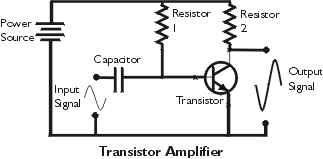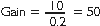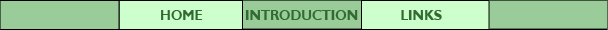## Part 3: AmplificationTransistors are often used as amplifiers to increase an input signal in radios, televisions, stereos and other similar applications. This field of electronics is known as linear electronics because there is a direct relationship between the strength of the input signal and the strength of the ouput signal. In the simple circuit shown above, the base is biased so that the transistor is always on. An increase or decrease in the input signal produces a corresponding decrease or increase in the output. The frequency of the output is the same as the input, but the polarity of the signal is inverted.

### Gain

The measure of amplification is the gain of the transistor. For example, if the input signal has an amplitude of 0.2 volts, and the output signal has an amplitude of 10 volts, then:The circuit may be designed to increase the amount of current (current gain) or the voltage level (voltage gain). The power gain is the product of current gain and voltage gain (P = V x I).